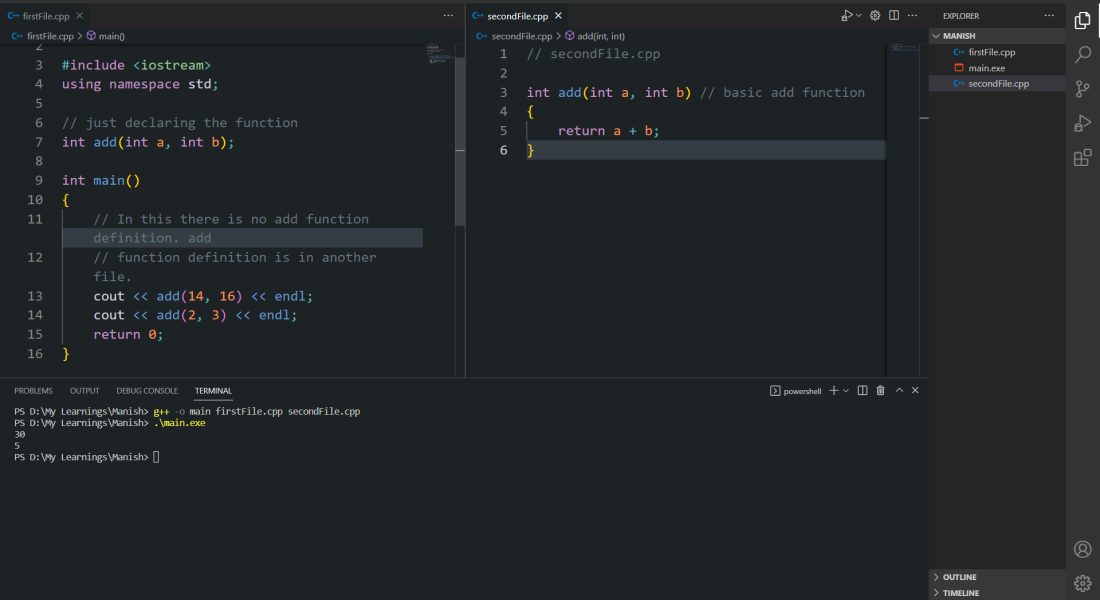Open in App
Not now

# Build a C++ Program that Have Multiple Source Code Files

• Difficulty Level : Medium
• Last Updated : 06 Feb, 2023

In C++ generally, we saw only a single file program but what if we have multiple source code files and we have to build a program by using all these files? Let’s see how we can build a C++ program with multiple source files without including them as header files.

## Use two or more source code files in C++

There are two methods to use two or more source code files in C++ as mentioned below:

2. Using -gcc commands

Example:

```#include<iostream>
// Here all basic commands can be used using this files```

So, how to use another file let us check with an example.

First Program:

## C++

 `// firstFile.h``// C++ Program to implement``// using gcc commands``int` `add(``int` `a, ``int` `b) { ``return` `a + b; }`

Second Program:

## C++

 `// secondFile.cpp``// C++ Program to implement``// Using gcc commands``#include ``#include "firstFile.h"``using` `namespace` `std;`` ` `// just declaring the function``int` `add(``int` `a, ``int` `b);`` ` `int` `main()``{``    ``// In this there is no add function definition. add``    ``// function definition is in another file.``    ``cout << add(14, 16) << endl;``    ``cout << add(2, 3) << endl;``    ``return` `0;``}`

Output:Output with the method with a header file

### 2. Using GCC Command to Build C++ Program

In this method, we will use the gcc command for running the program because of which we bind both the programs together while running. But, before running the program let’s check how C++ programs work.

### Working on C++ Program

Firstly we need to get an idea of how to compile a C++ program with GCC commands. It goes from the following steps :

• Preprocessing: only comments are removed from the source code and header files code is pasted in the file also macros are placed where they are used
• Compiling: texts are converted to machine code but function calls are not mapped with function definitions.
• Linking: the mapping between function calls and function definitions happens as its name suggests ‘linking’. It links the function calls and function definitions

At the linking phase, we have to add all the compiled C++ files (object files) so that the linker links all the files and executables can be formed.

#### Command to build a C file with more than one C source code file

g++ -o main firstFile.cpp secondFile.cpp

By using the above command we can build a C++ executable. We can add multiple files as arguments in the given command.

First Program:

## C++

 `// secondFile.cpp``// C++ Program to implement``// using gcc commands``int` `add(``int` `a, ``int` `b) { ``return` `a + b; }`

Second Program:

## C++

 `// firstFile.cpp``// C++ Program to implement``// Using gcc commands``#include ``using` `namespace` `std;`` ` `// just declaring the function``int` `add(``int` `a, ``int` `b);`` ` `int` `main()``{``    ``// In this there is no add function definition. add``    ``// function definition is in another file.``    ``cout << add(14, 16) << endl;``    ``cout << add(2, 3) << endl;``    ``return` `0;``}`

Demonstration:Output using GCC method

My Personal Notes arrow_drop_up